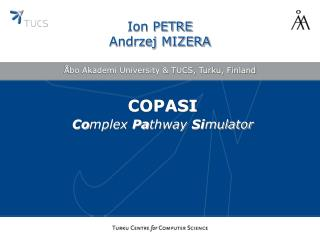DownloadDownload PresentationÅbo Akademi University & TUCS, Turku, Finland

# Åbo Akademi University & TUCS, Turku, Finland

Download Presentation## Åbo Akademi University & TUCS, Turku, Finland

- - - - - - - - - - - - - - - - - - - - - - - - - - - E N D - - - - - - - - - - - - - - - - - - - - - - - - - - -
##### Presentation Transcript

1. Ion PETREAndrzej MIZERA Åbo Akademi University & TUCS, Turku, Finland COPASI Complex Pathway Simulator

2. Outline • General information about COPASI • The Lotka-Volterra model of predator-prey interactions • Implementation of predator-prey model in COPASI • Time-course simulation, steady state analysis, parameter estimation in COPASI • Comments on numerical difficulties • Summary

3. General information on COPASI COPASI - Complex Pathway Simulator • Software application for simulation and analysis of biochemical networks • Free for non-commercial use • Features • stochastic and deterministic time course simulation • steady state analysis • metabolic control analysis/sensitivity analysis • parameter estimation using data from time course and/or steady state experiments • imports and exports SBML • http://www.copasi.org

4. Lotka-Volterra system • The Lotka-Volterra system of chemical reactions describes an ecological predator-prey (fox-rabbit)model. • chemical reactions: • growth of prey population: prey  2 prey • consumption of preys: predator + prey  predator • death of predators: predator   • increase of predator population: predator + prey  2 predator + prey

5. Lotka-Volterra system • The differential equations for the Lotka-Volterra system are obtained by applying the mass-action law. • The Lotka-Volterra system assumes that: • the prey population x grows at a rate proportional to the current population (Ax dt), • but when predators y are present, the prey populationdecreases at a rate proportional to the number of predator/prey encounters (–B x y dt); • the predator population declines at a rate proportional to the current population (–C y dt), • but increases at a rate proportional to the predator/prey meetings (D x y dt), where A, B, C, and D are positive constants.

6. Lotka-Volterra system • The differential equations for the Lotka-Volterra system:

7. Lotka-Volterra system • The system consists of two ordinary, non-linear, first order differential equations. • The equations have periodic solutions which do not have a simple expression in terms of the usual trigonometric functions. “No matter what the population of prey and predator are, neither species will die out, nor will its population grow indefinitely.” Differential Equations, Dynamical Systems, and an Introduction to Chaos, M. Hirsch, R. L. Devaney, S. Smale (2004) • In order to obtain time course graphs we need to use numerical integration.

8. Lotka-Volterra system An equilibrium solution is a set of concentrations/particle numbers which will not change over time, and hence can be found by solving the following set of equations: Solving it for x(t) and y(t) gives 2 solutions: and

9. stationary point Lotka-Volterra system An equilibrium solution is a set of concentrations/particle numbers which will not change over time, and hence can be found by solving the following set of equations: Solving it for x(t) and y(t) gives 2 solutions: and

10. How to implement a model in COPASI

11. Parameter estimation objective function value: 0.194162

12. Numerical difficulties ode23 ode45 ode113 predator: 20, prey: 20, A: 8.68876, B: 0.0872658, C: 8.3535, D: 0.168216

13. Numerical difficulties • COPASI does not solve analytically the differential equations, but integrates them with a certain numerical method. • Each numerical method has its own precision which in general strongly depends on the equations being solved. Usually we do not know the numerical properties of the system, hence do not know if a particular method is suitable for it. • Parameter Estimation task heavily utilizes the numerical integration to evaluate the fit. Thus, the obtained parameters depend not only on the experimental data but also on the chosen numerical method!

14. Summary • COPASI enables the user to implement a molecular model in a straightforward way by defining the chemical reactions in a simple format. • COPASI allows to perform both deterministic and stochastic simulations in an easy and fast way. • COPASI does not require any sophisticated knowledge of mathematics. It automatically generates mathematical model (differential equations) from the molecular model (chemical reactions). • COPASI implements a rich variety of parameter estimation methods. They are based on different heuristics and can be applied consecutively in any order – the next method starts with parameters estimated by the previous one.

15. Summary • COPASI provides a set of standard tools utilized in systems biology, e.g. Metabolic Control Analysis, Sensitivities and other.